# Latex公式编号: 多行公式多编号，多行公式单编号

多行公式单编号，编号居中

多行公式多编号，有的行没有编号

• \begin{align} 与 \begin{equation}不能同时使用
• \begin{aligned} 只能与 \begin{equation}同时使用
• 要想使用\begin{align}，需要在 \begin{document} 之前插入\usepackage{amsmath}
• \begin{align}的用法应该与\begin{gather}用法相同，区别是：
• \begin{align}是默认右对齐，或者用 & 指定对齐位置
• \begin{gather} 默认是居中对齐，不能与 & 结合使用
• 概括：

• 导言区要加上，amsmath宏包
• 如果想有编号，可以用gather，align
• 如果想某一行没有编号，在用\\换行前使用\nonumber
• 如果不想有编号，可以gather*，align*，或者在后面加上命令\notag
• 如果想一行公式分开写，用split
• 如果想选择判断，用cases
• align，split，cases 的公式对齐，用&
• 这里主要介绍使用 \begin{align}的公式编号，以LSTM的公式为例子：

## 多行公式多编号

% 多行公式多编号
\begin{align}
\hat{C}_t &= tanh(W_C\odot[h_{t-1},x_t]+b_C) \\
i_t&=\sigma(W_i\odot[h_{t-1},x_t]+b_i) \\
f_t&=\sigma(W_f\odot[h_{t-1},x_t]+b_f) \\
C_t&=f_t * C_{t-1}+i_t * \hat{C}_t \\
o_t&=\sigma(W_o\odot[h_{t-1},x_t]+b_o) \\
h_t&=o_t * tanh(C_t)
\end{align}

实现效果：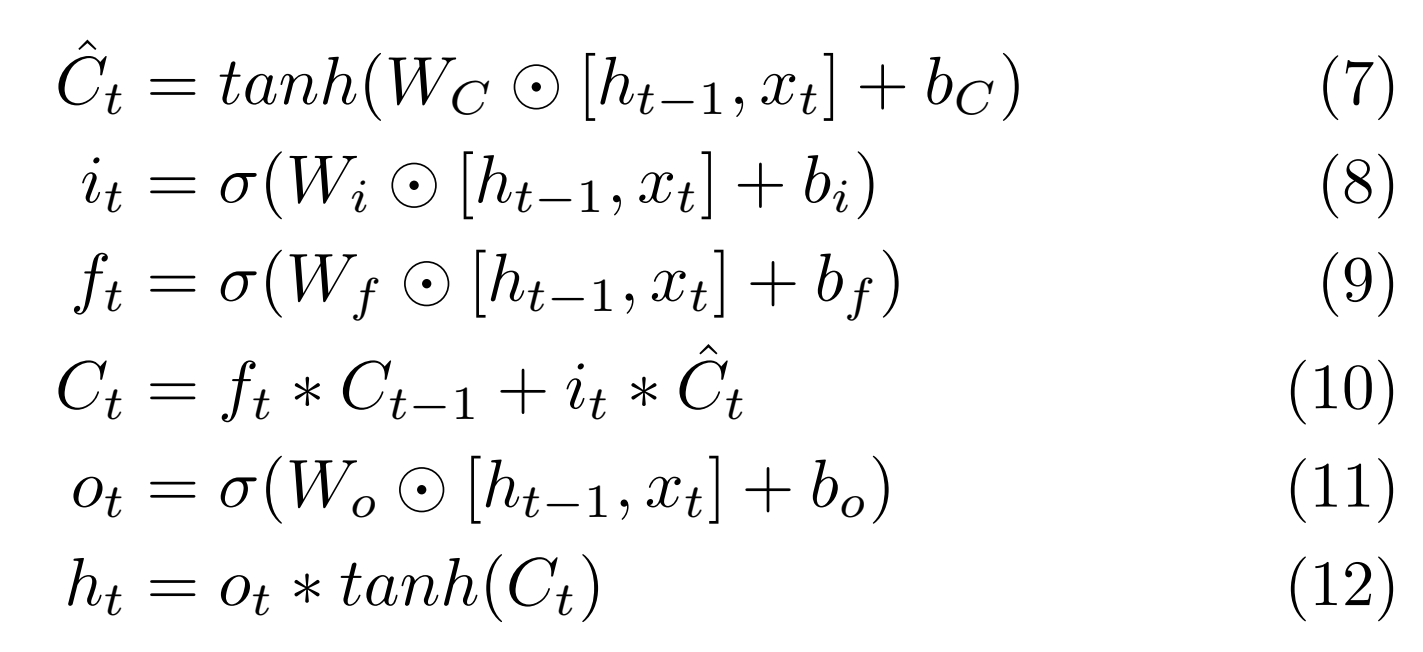## 多行公式单编号，编号居中

% 多行公式单编号
\begin{equation}
\begin{aligned}
&\hat{C}_t = tanh(W_C\odot[h_{t-1},x_t]+b_C) \\
&i_t=\sigma(W_i\odot[h_{t-1},x_t]+b_i) \\
&f_t=\sigma(W_f\odot[h_{t-1},x_t]+b_f) \\
&C_t=f_t * C_{t-1}+i_t * \hat{C}_t \\
&o_t=\sigma(W_o\odot[h_{t-1},x_t]+b_o) \\
&h_t=o_t * tanh(C_t)
\end{aligned}
\end{equation}

实现效果：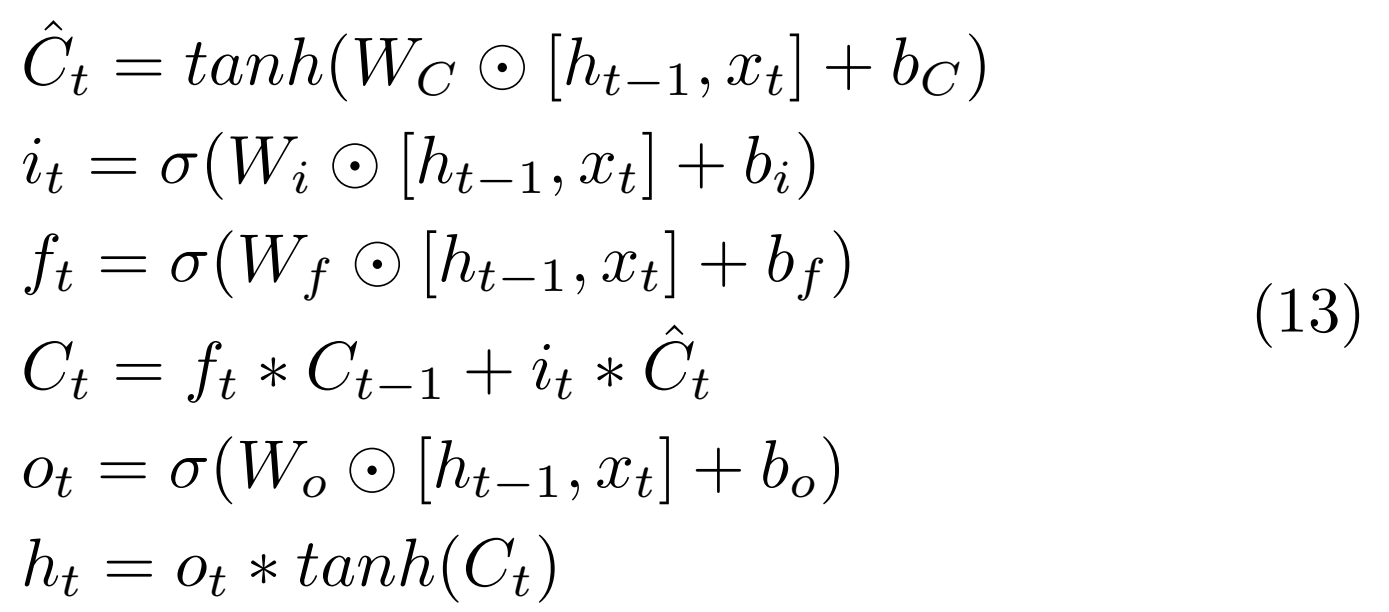## 多行公式无编号

% 多行公式无编号
\begin{align*}
\hat{C}_t &= tanh(W_C\odot[h_{t-1},x_t]+b_C) \\
i_t&=\sigma(W_i\odot[h_{t-1},x_t]+b_i) \\
f_t&=\sigma(W_f\odot[h_{t-1},x_t]+b_f) \\
C_t&=f_t * C_{t-1}+i_t * \hat{C}_t \\
o_t&=\sigma(W_o\odot[h_{t-1},x_t]+b_o) \\
h_t&=o_t * tanh(C_t)
\end{align*}

实现效果：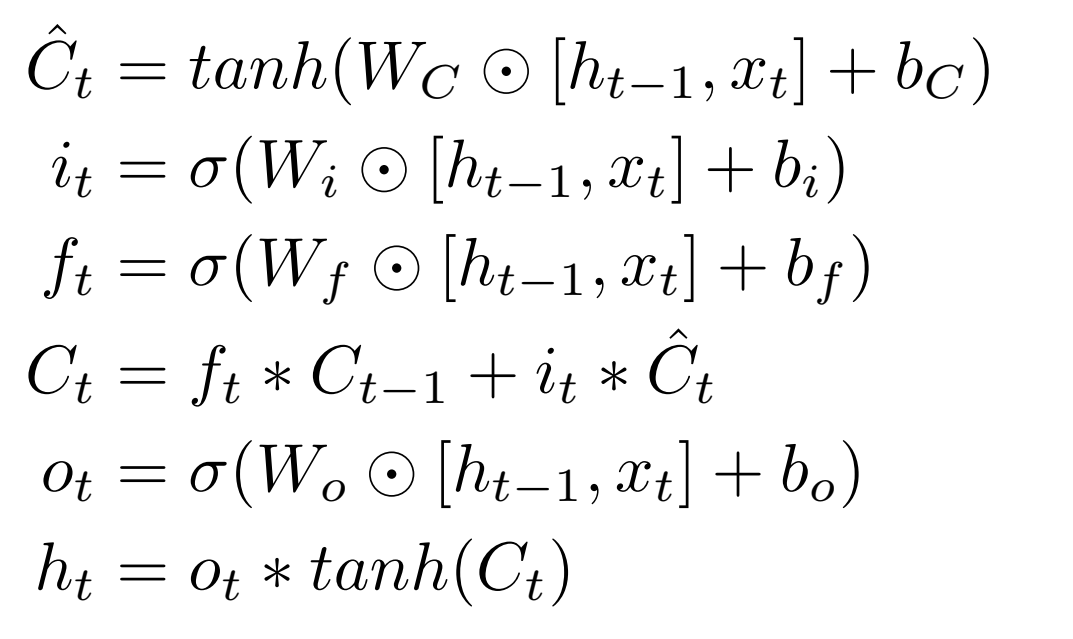## 多行公式多编号，有的行没有编号

% 多行公式多编号，有的行没有编号
\begin{align}
\hat{C}_t &= tanh(W_C\odot[h_{t-1},x_t]+b_C) \\
i_t&=\sigma(W_i\odot[h_{t-1},x_t]+b_i)  \nonumber \\
f_t&=\sigma(W_f\odot[h_{t-1},x_t]+b_f) \\
C_t&=f_t * C_{t-1}+i_t * \hat{C}_t \\
o_t&=\sigma(W_o\odot[h_{t-1},x_t]+b_o) \\
h_t&=o_t * tanh(C_t)
\end{align}

实现效果：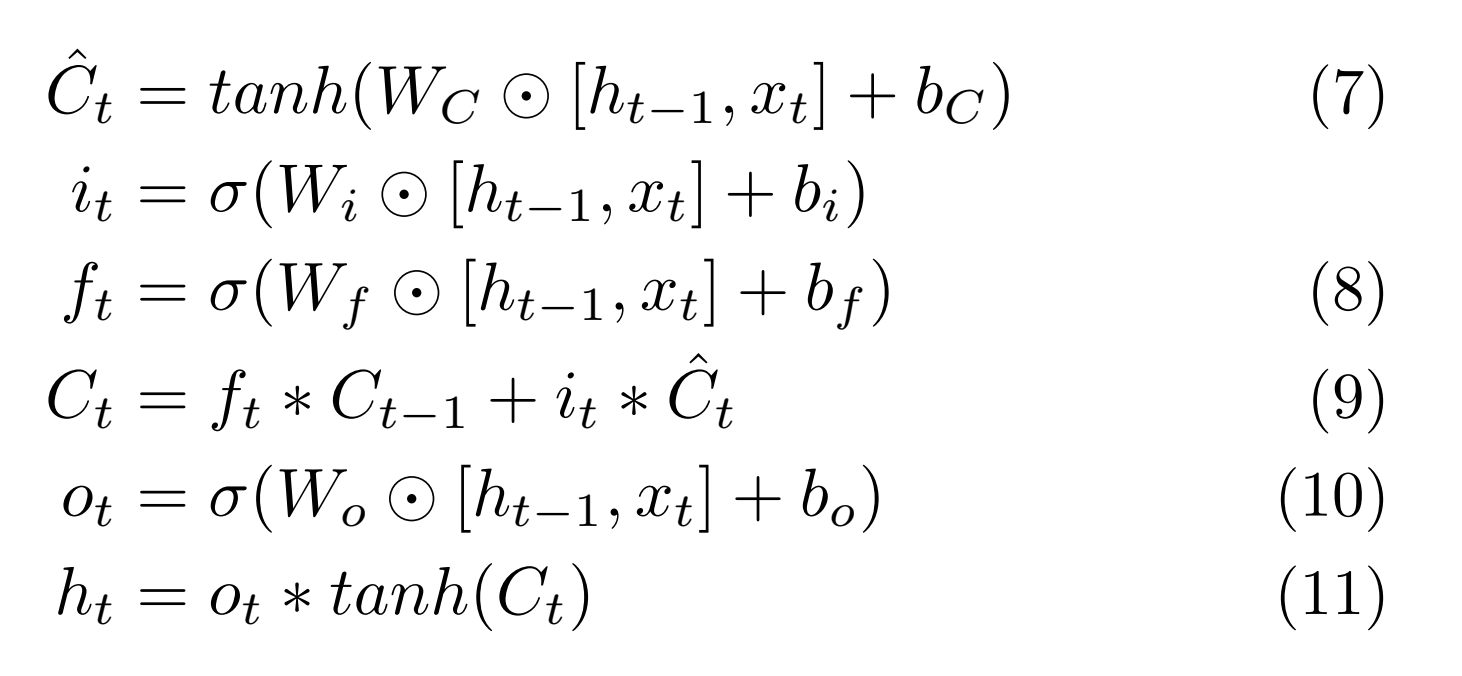## 一行公式分多行写

%split环境（用\$对齐）(一个公式分为多行排版)
\begin{equation}
\begin{split}
\cos 2x &= \cos^2 x - \sin^2 x\\
&= 2\cos^2 x - 1
\end{split}
\end{equation}

实现效果：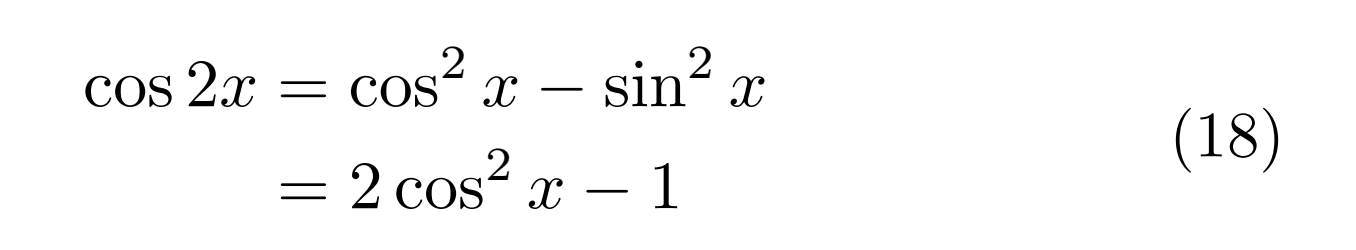## 情况（case）划分

\begin{equation}
D(x) = \begin{cases}
1, & if \ x \in Q \\
0, & if \ x \in R
\end{cases}
\end{equation}

实现效果：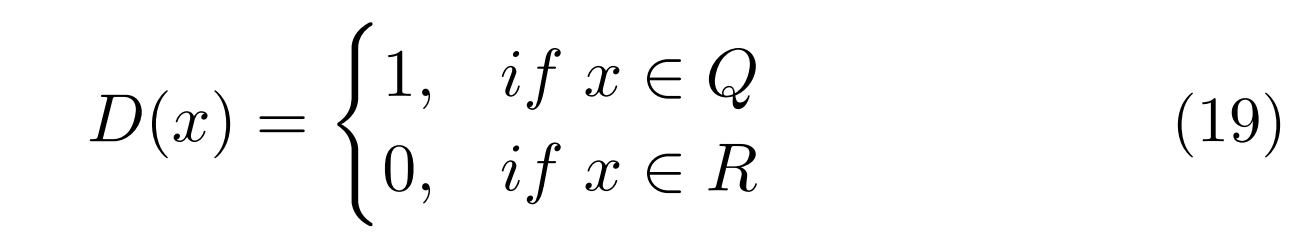## 大括号单编号

\begin{align}
\left\{     %在equation环境下使用，用\left\{命令添加左大括号，用\right.以打点.结束
\begin{aligned}
x&=eq1\\
y&=eq2+1
\end{aligned}
\right.
\end{align}

实现效果：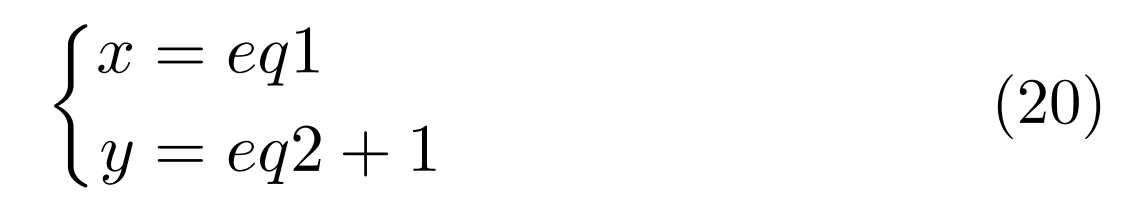## 大括号多编号

\usepackage{cases}

% 大括号多编号
\begin{numcases}{}
x_1&=eq1 \label{eqsystem1} \\
x_2+1&=eq2 \label{eqsystem2}
\end{numcases}

实现效果：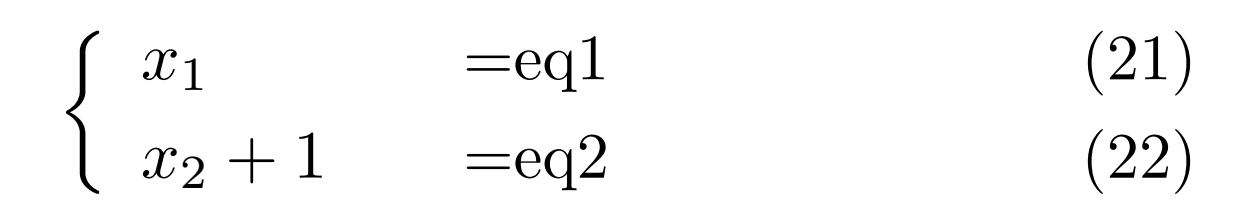更多大括号与编号的情况参考：latex多行公式加大括号、整体编号及多行编号及不同方法的区别

来源：wzg2016